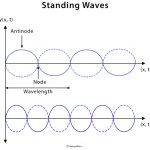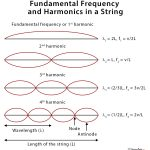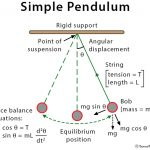Home / Physics / Fick’s Laws of Diffusion

# Fick’s Laws of Diffusion

## What are Fick’s Laws of Diffusion

Fick’s laws of diffusion are mathematical statements describing how particles under random thermal motion tend to spread from a region of higher concentration to a region of lower concentration to equalize concentration on both the regions. The laws also describe the relationship between the rate of diffusion and the three factors that affect diffusion.

They state that ‘the rate of diffusion is directly proportional to both the surface area and concentration difference and is inversely proportional to the thickness of the membrane’.

A diffusion process that obeys Fick’s laws is called normal or Fickian diffusion; otherwise, it is called anomalous diffusion or non-Fickian diffusion.

Thus, Fick’s laws can be mathematically written as:

Rate of Diffusion ∝ Surface area x Concentration gradient/Thickness of membrane

This means that the diffusion rate gets double if the surface area or concentration gradient is doubled, and the thickness of the membrane is halved.

### History and Background

They were developed by Adolf Fick in 1855 to mathematically represent the rate of diffusion of dissolved gases across membranes. Inspired from the early works of Thomas Graham, they dealt with measuring the concentrations and fluxes of salt, diffusing between two reservoirs through tubes of water. It is notable that Fick’s work primarily concerned diffusion in fluids. Today, Fick’s Laws form the core of our understanding of diffusion in solids, liquids, and gases except for the bulk fluid motion in the latter two cases.

## Fick’s First Law

It states that the movement of particles (diffusion flux) from a region of high concentration to a low concentration region is directly proportional to the magnitude of the concentration gradient of the substance.

The below-given equation of Fick’s first law is specific to one-dimensional objects.

The diffusion flux (J) measures the amount of substance that flows through a unit area during a unit time interval, measured in g/m2

The diffusion coefficient (D), measured in area per unit time m2/s. It is proportional to the squared velocity of the diffusing particles, which depends on the temperature, viscosity of the fluid, and the size of the particles. For biological molecules, the diffusion coefficients normally range from 10−11 to 10−10 m2/s.

The negative sign of the equation indicates that diffusion occurs in a direction opposite to that of the increasing concentration. Hence, diffusion occurs in the direction of decreasing concentration of the diffusing substance, and thus, the diffusion flux is a positive quantity.

For two or more dimensions, we use ‘∇’ the symbol of gradient which generalizes the first derivative as:

J = – Dφ

where,

J = diffusion flux vector

## Fick’s Second Law

It is derived from the first law, to predict how diffusion causes a change in concentration with respect to time.

The below-given equation of Fick’s second law is specific to one-dimensional objects.

For two or more dimensions, we use the Laplacian Δ = ∇2, which generalizes the second derivative, as:

This has the same mathematical form as the Heat equation except switching thermal conductivity ‘k‘ with diffusion coefficient ‘D‘.

## Fick’s Laws Explained with Examples

The principal of Fick’s laws can be practically illustrated by opening a perfume bottle in the corner of a closed room. After a certain time, the room is found to be filled with the perfume odor. This occurs because the perfume molecules have diffused from one side of the room to the other, from a region of high concentration to a region of low concentration to attain a state of equilibrium or become even throughout.

## Applications of Fick’s Law

Biological Applications

1) Fick’s First Law gives rise to the formulae:

Diffusion Flux = -P (c2-c1),

where,

P is the permeability, an experimentally determined membrane ‘conductance’ for a given gas at a given temperature

c2-c1 is the difference in concentration of the gas across the membrane for the direction of flow from c1 to c2

2) Applicable to explain diffusion for two miscible liquids when they are brought in contact since the diffusion occurs at a macroscopic level.

3) Fick’s first law is also important in radiation transfer equations. However, in this context, it becomes inaccurate when the diffusion constant is low, and the radiation becomes limited by the speed of light rather than by the resistance of the material the radiation is flowing through. In such situation a flux limiter is used.

4) The exchange rate of oxygen and carbon dioxide in the lungs across the alveolar membrane can be determined using the Fick’s first law and Graham’s law.

Industrial Applications

1) Used to model transport processes in foods, neurons, biopolymers, pharmaceuticals, porous soils, population dynamics, nuclear materials, plasma physics, and semiconductor doping processes.

2) Integrated circuit fabrication technologies, model processes like CVD, thermal oxidation, wet oxidation, and doping, use diffusion equations obtained from Fick’s laws.

Article was last reviewed on Saturday, July 11, 2020

### Related articlesStanding WavesFundamental FrequencySimple PendulumKirchhoff’s Law